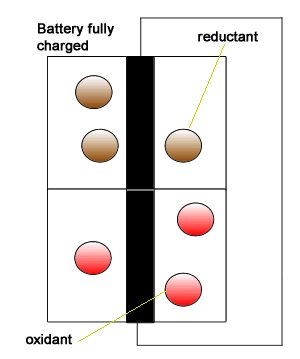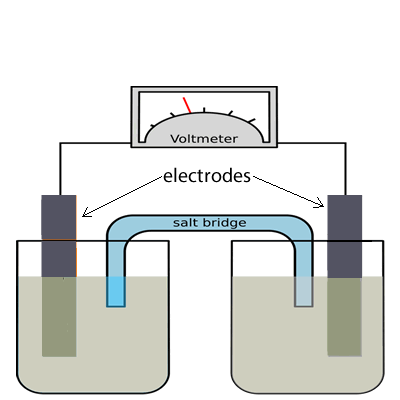Constructing an electrochemical cell from an overall equation For beginners Initially we introduced the battery as having two isolated compartments connected by an external wire through which electrons flow. This simplistic view is pictured on the right. This representation shows the oxidant (electron taker) and the reductant (electron giver) exchanging electrons and building up positive and negative charge. In reality this can not be allowed to happen inside the battery as electron flow will immediately cease. A salt bridge is used to prevent a build up of charge by supplying positive and negative ions to each chamber of the battery . Click to see a simple representation of how the salt bridge works.1) The image on the right shows the standard representation of a galvanic cell. A galvanic cell is an apparatus where redox reactions take place to convert chemical energy into electrical energy. Any redox reaction can be used to produce electrons in a galvanic cell. The trick is to separate the oxidant and reductant into the separate chambers (half-cells) of the galvanic cell. For example, consider the metal displacement reaction below. Zn(s) + Cu2+(aq) =>Cu(s) + Zn2+(aq) Follow the steps below Step 1 Identify the oxidant and reductant on the reactants side. Step 2 Using the electrochemical series see if the oxidant is above the reductant, in other words, will a spontaneous reaction occur. Step 3 If a spontaneous reaction  will take place then write the two conjugate pairs. Step 4 Place each conjugate pair in a separate half-cell of the galvanic cell. If one of the conjugates is a metal use it as an electrode otherwise use platinum or carbon electrodes. Step 5 Write the oxidation half equation in the half-cell of the reductant and the reduction half equation in the half cell of the oxidant. Step 6 Electrons always flow from the oxidation half-cell to the half-cell where reduction takes place. While negative ions from the salt bridge always flow into the half-cell where oxidation is taking place. The electrode where oxidation takes place is known as the anode and has a negative polarity while the electrode where reduction takes place is known as the cathode and has a positive polarity. Below are 5 rules you should always apply to a galvanic cell. - anode is negative and is the electrode where oxidation takes   place - cathode is positive and it is the electrode where reduction takes place. - electrons always flow from anode to cathode. - negative ions, from the salt bridge towards the anode. - positive ions from the slat bridge towards the cathode.Consider the following overall equations. Identify if each one represents a sponatneous redox reaction and if so construct a fully labelled galvanic cell. a. Cu(s) + 2Ag+(aq) => 2Ag(s) + Cu2+(aq)        Solution b.Fe(s) + 2Ag+(aq) => 2Ag(s) + Fe2+(aq)          Solution c. Zn(s) + Sn4+(aq) => Zn2+(aq)+ Sn2+(aq)         Solution d) Ag(s) + Al3+(aq) => Ag+(aq)+ Al(s)                 Solution e) Pb(s) + 2Fe3+(aq) =>Pb2+(aq)+ 2Fe2+(aq)   Solution f) Cl2(s) + Sn2+(aq) =>2Cl-(aq)+ Sn4+(aq           SolutionContinue